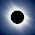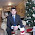# How to swap two Integers without using temporary variable in Java?

One of the oldest trick question from programming interview is, How do you swap two integers without using temp variable? This was first asked to me on a C, C++ interview and then several times on various Java interviews. Beauty of this question lies both on trick to think about how you can swap two numbers with out third variable, but also problems associated with each approach. If a programmer can think about integer overflow and consider that in its solution then it creates a very good impression in the eye of interviewers. Ok, so let's come to the point, suppose you have tow integers i = 10 and j = 20, how will you swap them without using any variable so that j = 10 and i = 20? Though this is journal problem and solution work more or less in every other programming language, you need to do this using Java programming constructs. You can swap numbers by performing some mathematical operations e.g. addition and subtraction and multiplication and division, but they face problem of integer overflow.

There is a neat trick of swapping number using XOR bitwise operator which proves to be ultimate solution. We will explore and understand each of these three solutions in detail here, and if you are hungry for more programming questions and solutions, you can always take a look at Cracking the Coding Interview: 150 Programming Questions and Solutions, one of the best book to prepare for programming job interviews.

### Solution 1 - Using Addition and Subtraction

You can use + and - operator in Java to swap two integers as shown below :
```a = a + b;
b = a - b;   // actually (a + b) - (b), so now b is equal to a
a = a - b;   // (a + b) -(a), now a is equal to b```

You can see that its really nice trick and first time it took sometime to think about this approach. I was really happy to even think about this solution because its neat, but happiness was short lived because interviewer said that it will not work in all conditions. He said that integer will overflow if addition is more than maximum value of int primitive as defined by Integer.MAX_VALUE and if subtraction is less than minimum value i.e. Integer.MIN_VALUE.

It confused me and I conceded only to find that the code is absolutely fine and work perfectly in Java, because overflows are clearly defined. Ofcourse same solution will not work in C or C++ but it will work absolutely fine in Java. Don't believe me? here is the proof :

```a = Integer.MAX_VALUE;
b = 10;

System.out.printf("Before swap 'a': %d, 'b': %d %n", a, b);

a = (a + b) - (b = a);

System.out.printf("After swapping, 'a': %d, 'b': %d %n", a, b);

Output :
Before swap 'a': 2147483647, 'b': 10
After swapping, 'a': 10, 'b': 2147483647 ```

Here addition of two numbers will overflow, Integer.MAX_VALUE + 10 = -2147483639, but we are also doing subtraction, which will compensate this value, because b is now Integer.MAX_VALUE and -2147483639 - 2147483647 will again overflow to give you 10 as output.

### Solution 2 - Using XOR trick

Second solution to swap two integer numbers in Java without using temp variable is widely recognized as the best solution, as it will also work in language which doesn't handle integer overflow like Java e.g. C and C++. Java provides several bitwise and bitshift operator, one of them is XOR which is denoted by ^.

If you remember XOR truth table then you know that A XOR B will return 1 if A and B are different and 0 if A and B are same. This property gives birth to following code, popularly know as XOR trick:

```x = x ^ y;
y = x ^ y;   // now y = x
x = x ^ y;  // now x = y```

Let's see these examples in action by writing a simple Java program, as shown below.

## Progarm to Swap Two Integers without tempoarry variable in Java

In this Java program, I will show you couple of ways to swap two integers without using any temporary or third variable, and what are problems comes with each approach and which one will work in all conditions. Actually the two popular solution works perfectly fine in Java but candidates, especially those coming from C and C++ background often think that first solution is broken and will fail on integer boundaries, but that's not true. Integer overflows are clearly define in Java e.g. Integer.MAX_VALUE + 1 will result in Integer.MIN_VALUE, which means if you do both addition and subtraction in with same set of numbers, your result will be fine, as seen in above example.

```/**
* Java program to swap two integers without using temp variable
*
* @author java67
*/
public class Problem {

public static void main(String args[]) {
int a = 10;
int b = 20;

// one way using arithmetic operator e.g. + or -
// won't work if sum overflows
System.out.println("One way to swap two numbers without temp variable");
System.out.printf("Before swap 'a': %d, 'b': %d %n", a, b);
a = a + b;
b = a - b; // actually (a + b) - (b), so now b is equal to a
a = a - b; // (a + b) -(a), now a is equal to b

System.out.printf("After swapping, 'a': %d, 'b': %d %n", a, b);

// another example
a = Integer.MIN_VALUE;
b = Integer.MAX_VALUE;

System.out.printf("Before swap 'a': %d, 'b': %d %n", a, b);

a = (a + b) - (b = a);

System.out.printf("After swapping, 'a': %d, 'b': %d %n", a, b);

// Another way to swap integers without using temp variable is
// by using XOR bitwise operator
// Known as XOR trick
System.out.println("Swap two integers without third variable
using XOR bitwise Operator");

int x = 30;
int y = 60;

System.out.printf("Before swap 'x': %d, 'y': %d %n", x, y);
x = x ^ y;
y = x ^ y;
x = x ^ y;

System.out.printf("After swapping, 'x': %d, 'y': %d %n", x, y);
}

}

Output
One way to swap two numbers without temp variable
Before swap 'a': 10, 'b': 20
After swapping, 'a': 20, 'b': 10
Before swap 'a': -2147483648, 'b': 2147483647
After swapping, 'a': 2147483647, 'b': -2147483648
Swap two integers without third variable using XOR bitwise Operator
Before swap 'x': 30, 'y': 60
After swapping, 'x': 60, 'y': 30```

That's all about how to swap two integers without using temporary variable in Java. Unlike popular belief that first solution will not work on integer boundaries i.e. around maximum and minimum values, which is also true for languages like C and C++, it work perfectly fine in Java. Why? because overflow is clearly define and addition and subtraction together negate the effect of overflow. There is no doubt about second solution as it is the best solution and not subject to any sign or overflow problem. It is also believed to be fasted way to swap two numbers without using temp variable in Java.

and how about this meme :)

If you are interested in solving some simple and some tricky programming questions, I suggest you to take a look at following set of programming problems :
• Top 30 Array based programming problems for Java programmers? [problems]
• Top 20 String based coding problems for Java programmers? [problems]
• Top 10 Programming and Coding exercises for Java programmers? [problems]
• How to find top two numbers from integer array? [solution]
• How to find duplicate characters in Java String? [solution]
• How to find square root of a number in Java? [solution]
• How to print Fibonacci series in Java without using recursion? [solution]

If you are preparing for programming job interviews and looking for some good resources, then you can also take help from following books, two of the best resources of programming questions :
• Programming Interviews Exposed: Secrets to Landing Your Next Job [see here]
• Cracking the Coding Interview: 150 Programming Questions and Solutions [see here]

Further Learning
The Coding Interview Bootcamp: Algorithms + Data Structures
Data Structures and Algorithms: Deep Dive Using Java
Algorithms and Data Structures - Part 1 and 2

#### 11 comments:

1.How does the XOR swap hold up when both x and y are the same number? ;)

1.XOR swap holds up just fine when x and y are the same number.

x ^ x is always 0. 0 ^ x is always x. soo...
if x == y
x = x ^ x
y = 0 ^ y
x = 0 ^ y

with real numbers
x = 5 and y = 5
x = 5 ^ 5 = 0
y = 0 ^ 5 = 5
x = 0 ^ 5 = 5

2.It fails, however, if x and y are both references to the _same variable_ (such as might happen if passed as reference parameters to a function).

It devolves to:

x ^ x = 0;
0 ^ 0 = 0;
0 ^ 0 = 0;

// therefore x = y = 0, regardless of input.

3.Hello Keith, it did work well in Java. I am not sure which specific case you are referring, because int primitive are immutable in Java and they are also shared.

here is the code snippet from Java:

public class HelloWorldApp {

public static void main(String args[]) {
int x = 3;
int y = 3;

System.out.println("before swapping x: " + x);
System.out.println("before swapping y: " + x);

x = x ^ y;
y = x ^ y; // now y = x
x = x ^ y; // now x = y

System.out.println("after swapping x: " + x);
System.out.println("after swapping y: " + x);
}
}
Output
before swapping x: 3
before swapping y: 3
after swapping x: 3
after swapping y: 3

2.a = 10 and b = 20.

a = a * b = 200
b = a/b = 10 (swapped)
a = a/b = 20 (swapped)

1.Once again you end up with overflow (min/max negate the effect) I hope xor is the best solution

2.and breaks when a or b are zero (if b is zero then you get a /0 error; if a is zero then a*b == 0 and you can't get it back, and b gets assigned 0 and you get another /0 in the last line).

3.While this being a fun mathematical puzzle, I really don't get why it would be a good interview question. Such code will be understood by nobody else (i.e. it won't be maintainable) and probably quite a bit slower than using a temporary variable.

1.... and break in weird, inobvious ways

2.Totally agree!

4.Another cheap way to do it, if the integers are "small":

int BIG=1000;

int a = 7;
int b = 4;

System.out.println ("a is " + a + " and b is " + b);

a = a * BIG + b;
b = a / BIG;
a = a % BIG;

System.out.println ("a is " + a + " and b is " + b);